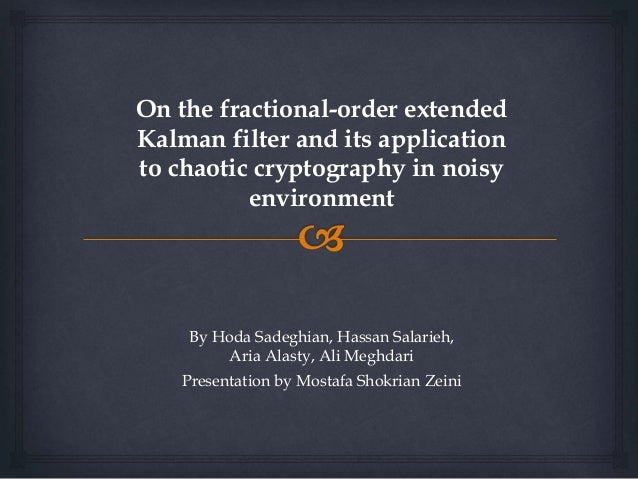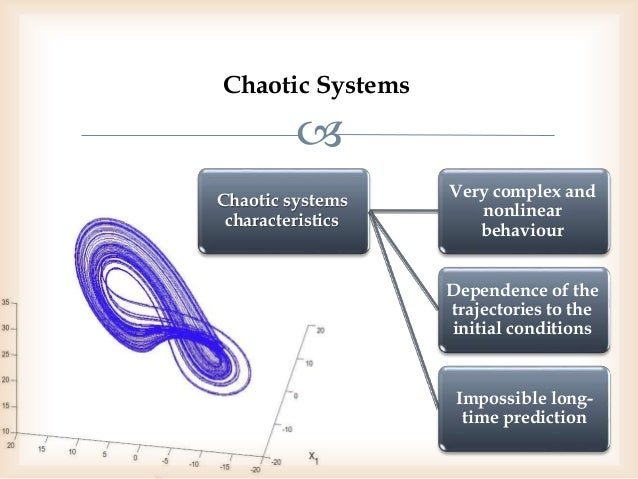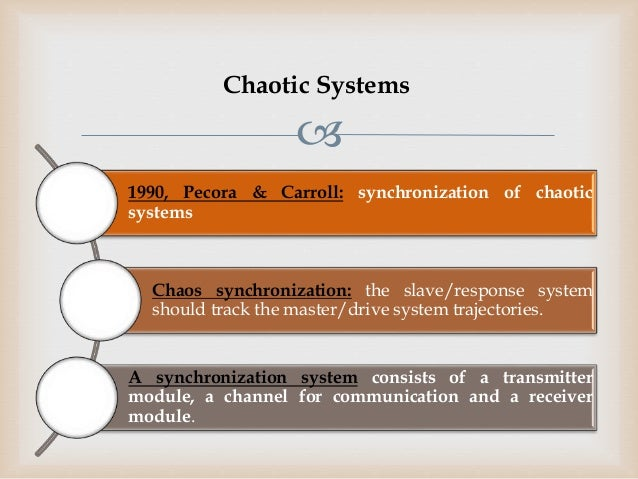Successfully reported this slideshow.Upcoming SlideShare
×

ofUpcoming SlideShare
Fractional Factorial Designs
Next

0

Share

# On the fractional order extended kalman filter and its application to chaotic cryptography in noisy environment

Reference:
H. Sadeghian, H. Salarieh, A. Alasty, A. Meghdari. "On the Fractional-Order Extended Kalman Filter and its Application to Chaotic Cryptography in Noisy Environment" Applied Mathematical Modelling, 2014, 38, pp. 961-973.

See all

### Related Audiobooks

#### Free with a 30 day trial from Scribd

See all
• Be the first to like this

### On the fractional order extended kalman filter and its application to chaotic cryptography in noisy environment

1. 1. On the fractional-order extended Kalman filter and its application to chaotic cryptography in noisy environment By Hoda Sadeghian, Hassan Salarieh, Aria Alasty, Ali Meghdari Presentation by Mostafa Shokrian Zeini
2. 2.  Chaotic Systems Chaotic systems characteristics Very complex and nonlinear behaviour Dependence of the trajectories to the initial conditions Impossible long- time prediction
3. 3.  1990, Pecora & Carroll: synchronization of chaotic systems Chaos synchronization: the slave/response system should track the master/drive system trajectories. A synchronization system consists of a transmitter module, a channel for communication and a receiver module. Chaotic Systems
4. 4.  -Application: *secure communication Chaotic Cryptography
5. 5.  BUT •Most of the works in chaotic communication have modeled the chaotic systems in deterministic form. IN THIS CASE •in real world applications due to random uncertainties such as stochastic forces on physical systems and noisy measurements caused by environmental uncertainties, a stochastic chaotic behavior is produced instead of a deterministic one. the deterministic differential equation of a system must be substituted by a stochastic one. Chaotic Communication
6. 6.  As to increase the complexity of the transmitter, one may use a fractional-order stochastic chaotic system as transmitter. A chaotic fractional-order dynamical equation produces a complex behavior which makes the masked signal more encrypted and consequently hard to decipher. The complexity of fractional-order systems is due to dealing with integration and derivation of non-integer orders. Fractional-order Stochastic Chaotic Systems
7. 7.  Kalman Filter for Linear Fractional-order Stochastic Systems Lemma
8. 8.  Then the Kalman filter can be obtained via following steps Kalman Filter for Linear Fractional-order Stochastic Systems
9. 9.  d. Updating equation c. Kalman gain equation b. Innovation equation a. Output prediction equation Kalman Filter for Linear Fractional-order Stochastic Systems: Design & Calculations
10. 10.  and where e. State prediction equation Kalman Filter for Linear Fractional-order Stochastic Systems: Design & Calculations
11. 11.  Then the Kalman filter can be obtained via following steps Extended Kalman Filter for Non-linear Fractional-order Stochastic Systems
12. 12.  c. Kalman gain equation b. Innovation equation where a. Output prediction equation Extended Kalman Filter for Non-linear Fractional-order Stochastic Systems: Design & Calculations
13. 13.  where where the initial condition assumed to be at time e. State prediction equation d. Updating equation Extended Kalman Filter for Non-linear Fractional-order Stochastic Systems: Design & Calculations
14. 14.  Except 𝛼 = 1, 𝜔 𝑘 is not a Wiener process and thus the Kalman filter presented here is dealing with non-Wiener process. The above theorems can be easily verified in the case of 𝛼 = 1 to be exactly equal to the classical Kalman and extended Kalman filter. FKF Design Remarks
15. 15.  while the output of the communication channel would be The output/measurement of this system can be assumed as The Caputo derivative of fractional order is defined as Let assume the transmitter module as a fractional-order stochastic chaotic system be Cryptography and Synchronization
16. 16.  To decrypt the message in the receiver, assuming the synchronization via nonlinear filtering has been done The noise of communication channel would change this output in the form of Let now assume the transmitter module has got another output with embedded message that should be encrypted. Cryptography and Synchronization
17. 17.  Cryptography and Synchronization
18. 18.  Using fractional-order Chen system for chaotic fractional-order cryptography
19. 19.  Fractional- order Chen system the output of noisy channel Using fractional-order Chen system for chaotic fractional-order cryptography where
20. 20.  Using fractional-order Chen system for chaotic fractional-order cryptography The first synchronized state of the fractional-order stochastic Chen chaotic system and its estimated error
21. 21.  Using fractional-order Chen system for chaotic fractional-order cryptography The second synchronized state of the fractional-order stochastic Chen chaotic system and its estimated error
22. 22.  Using fractional-order Chen system for chaotic fractional-order cryptography The third synchronized state of the fractional-order stochastic Chen chaotic system and its estimated error
23. 23.  The embedded message signal The output for encryption Using fractional-order Chen system for chaotic fractional-order cryptography where
24. 24.  Using fractional-order Chen system for chaotic fractional-order cryptography Encryption/Decryption of signal via fractional-order synchronization in the fractional-order stochastic Chen chaotic system and its estimated error

Total views

645

On Slideshare

0

From embeds

0

Number of embeds

3

39

Shares

0